# Potential Acoustic Gain

For a simple sound reinforcement system where the sound is assumed to drop off according to the inverse square law and the microphones and speakers are assumed to be omnidirectional, then the maximum achievable gain is determined by strictly geometric considerations. This simple case provides the framework for assessing what can be done in a real auditorium to increase the possible gain before feedback takes control.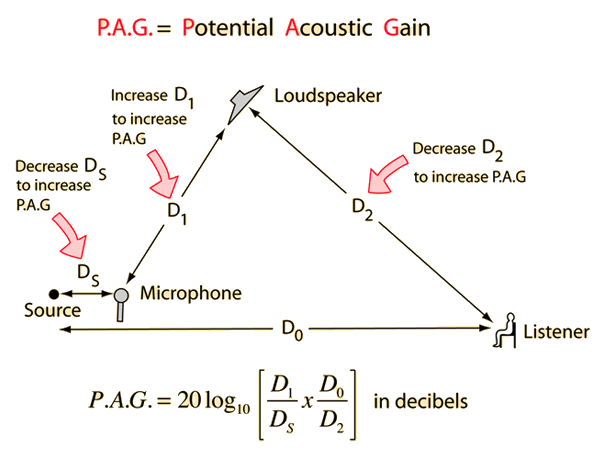Calculation Derivation of expression
 More detail about how to increase P.A. G.
Index

Sound reproduction concepts

 HyperPhysics***** Sound R Nave
Go Back

# Developing the Potential Acoustic Gain Expression

The maximum amplified level at the listener is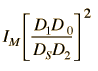Show

so the maximum gain in decibels is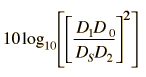and bringing out the exponent gives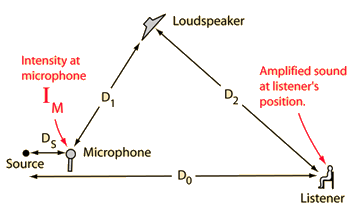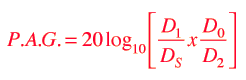Calculate
Index

Sound reproduction concepts

 HyperPhysics***** Sound R Nave
Go Back

# P.A.G. Expression Development

 According to the inverse square law, the sound intensity at the listener without amplification is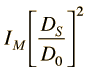and level at the listener which corresponds to giving a feedback level equal to the input is shown below.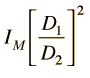This inverse square law expression gives the level at the listener if the loudspeaker is returning exactly the same level IM to the microphone that was originally produced at the microphone by the sound source. That is, it is returning exactly as much as it got as input so that there is a closed loop of signal returning to the microphone.
 The ratio of these two levels is the maximum gain before reaching the feedback condition. This ratio is used to develop the P.A.G. expression.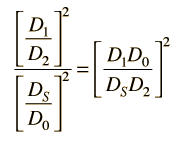Potential Acoustic Gain Calculation
Index

Sound reproduction concepts

 HyperPhysics***** Sound R Nave
Go Back

# Potential Acoustic Gain Calculation

For distance values

 DS =m=ft

 D1 =m=ft

 D0 =m=ft

 D2 =m=ft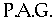= dBYou may enter values for the relevant distances in the simplified sound amplification system. Unspecified values will default to the values in the example model. You can change any values to convince yourself that the P.A.G. can be increased by increasing the distance from microphone to loudspeaker. The gain can also be increased by decreasing the distance from loudspeaker to listener or the distance from the source to the microphone.

 Simplified Amplification System Potential Acoustic Gain
Index

Sound reproduction concepts

 HyperPhysics***** Sound R Nave
Go Back